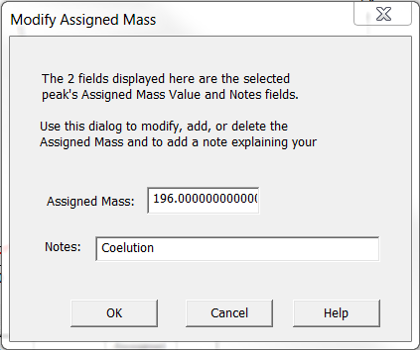# How to use peak tracking - WKB59742

Article number: 59742

## OBJECTIVE or GOAL

Welcome back to Get Empowered! In the last Empower tip-of-the-week post for Empower Chromatography Data Software, we learned about the benefits of smoothing a chromatogram using the Processing Method versus smoothing using a Derived Channel in the Method Set (Tip #108).

Working with ACQUITY QDa MS data in Empower:

• #100: How to view the base mass and mass spectra for the peaks in a chromatogram
• #101: How to work with 2D ACQUITY QDa MS data
• #103: Viewing different spectral views in the Mass Analysis window
• #104: Extracting chromatograms and spectra while working in the Mass Analysis window
• #105:  Why it is important to align chromatograms when collecting data from both a PDA and a QDa mass detector
• #106: How to optimize sensitivity by extracting a single mass rather than working with a TIC plot
• #107:  How to smooth the chromatogram using the Processing Method
• #108: How to smooth the chromatogram using a Derived Channel in the Method Set

This week we are going to learn about peak tracking. A typical reversed phase method development scenario would include trying different columns and varying the strong solvent for the mobile phase.  The challenge is tracking the peaks from experiment to experiment.  We could build a library of UV spectra for our compounds of interest with a PDA and then have Empower match the apex spectrum of each peak to the spectra in the library.  That works well if the UV spectra are sufficiently different for the compounds of interest.  An alternate approach would be to track the peaks by m/z ratio using the ACQUITY QDa mass detector.  We can then summarize peak tracking using Assigned Mass.

Let’s get started.

• Empower

## PROCEDURE

1. On the MS 3D Channel tab of the Processing Method, peak tracking is enabled by first selecting ‘Apex’ or ‘Combined’ from the Spectra for Assigned Mass drop down list (figure 1).  (Refer to Tip #103 for an explanation of ‘Combined’)Figure1

1. Set the desired precision (figure 2).Figure 2

1. Save the changes to the Processing Method and process the Sample Set.   Create a ‘Summary By All Report Method’ that includes the Peak Track Component Summary table (figure 3).Figure 3

1. This summarizes the peaks by Assigned Mass. We can track the peaks as we vary the chromatographic conditions.  For example, we see mass ‘182’ and the retention time for the peak with that mass over eight experiments (figure 4).Figure 4

1. Now let’s look at the Result Set. In the first result, we see the ‘Assigned Mass’, ‘Assigned Mass Value’, and the ‘Notes’ fields have been added to the Peaks table (figure 5).  The Base Peak value from either the Apex Spectrum or the Combined Spectrum is then copied into the ‘Assigned Mass Value’ field using the precision set in the Processing Method.Figure 5

1. In this result, we see that peaks 6 & 7 are fused and have the same Assigned Mass.  Empower has appended these Assigned Masses with numbers (figure 6).  Now let’s evaluate the assignment.Figure 6

1. In the Mass Analysis window, we look at the Purity View for these two peaks. (Refer to Tip# 103 for an explanation of Purity view). We may decide that the peak at 2.483 minutes has a mass of 196 and that the 202 comes from the peak at 2.465 minutes (figure 7).Figure 7

1. Back in the main window, ‘right click’ on the row for the peak 2.483 minutes and select ‘Modify Assigned Mass’ (figure 8).Figure 8

Enter the mass and any notes, then click OK (figure 9).Figure 9

The peak table has been updated (figure 10).  Save the result.Figure 10

1. Summarizing the Result Set once again, we see the new summary which includes the manual assignment (figure 11).Figure 11

It’s that easy!